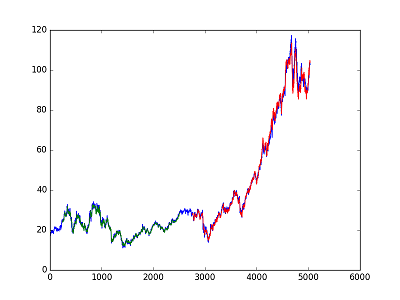# Prediction of Stock Prices Using LSTM network

Stock and ETFs prices are predicted using LSTM network (Keras-Tensorflow).

• Closed value (column) is used in the network.
• Values are normalized in range (0,1).
• Datasets are splitted into train and test sets, 50% test data, 50% training data.
• Keras-Tensorflow is used for implementation.
• LSTM network consists of 25 hidden neurons, and 1 output layer (1 dense layer).
• LSTM network features input: 1 layer, output: 1 layer , hidden: 25 neurons, optimizer:adam, dropout:0.1, timestep:240, batchsize:240, epochs:1000 (features can be further optimized).
• Root mean squared errors are calculated.
• Output files: lstm_results (consists of prediction and actual values), plot file (actual and prediction values).What is LSTM? (General Information) https://en.wikipedia.org/wiki/Long_short-term_memory

Keras: https://keras.io/

Tensorflow: https://www.tensorflow.org/